# Impermanent Loss for Standard AMM Pools

To better understand how to calculate Impermanent Loss (we’ll use the IL notation) in Uniswap V3, let’s first remember what it is for standard liquidity pools.
Such pools follow two basic rules:

1. They are composed of two assets (ETH-DAI, BTC-USDT…)
2. The valuation of the two assets represents 50% of the pool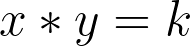(1)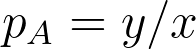(2) the price of asset A in terms of asset B, according to the poolImpermanent Loss for V2 pools, in %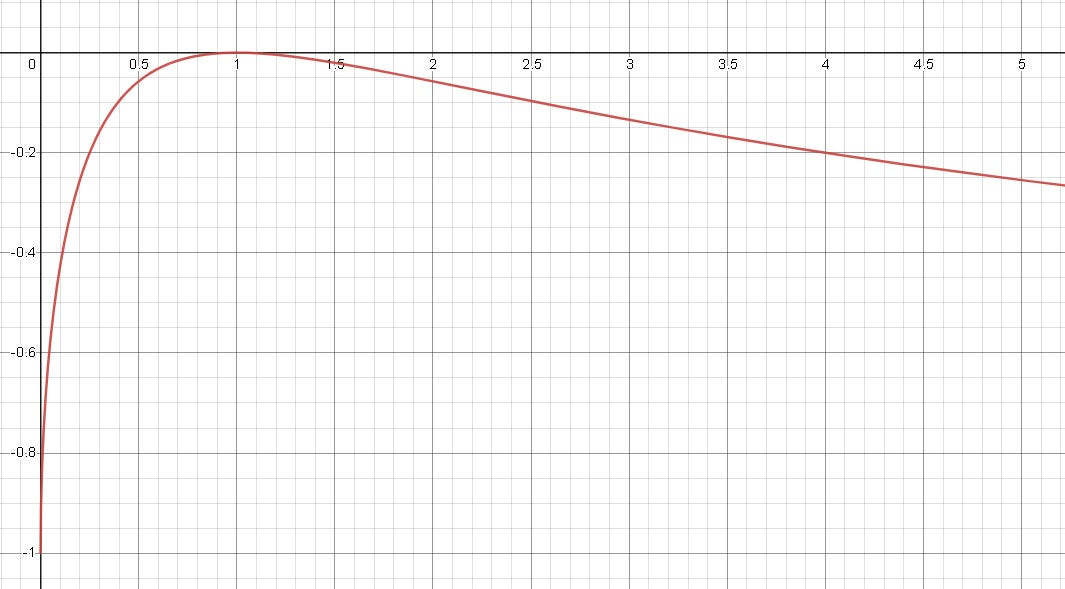Impermanent Loss for Standard Pools (Uniswap V2)

# Impermanent Loss for Uniswap V3 pools

The V3 of Uniswap introduces a new concept of concentrated liquidity. It means that the liquidity provider no longer deposits its liquidity for the entire price range of the assets, but only for a specific interval. The resulting V3 liquidity pool can be seen as a set of liquidity pools that are only valid for the range in which they are defined.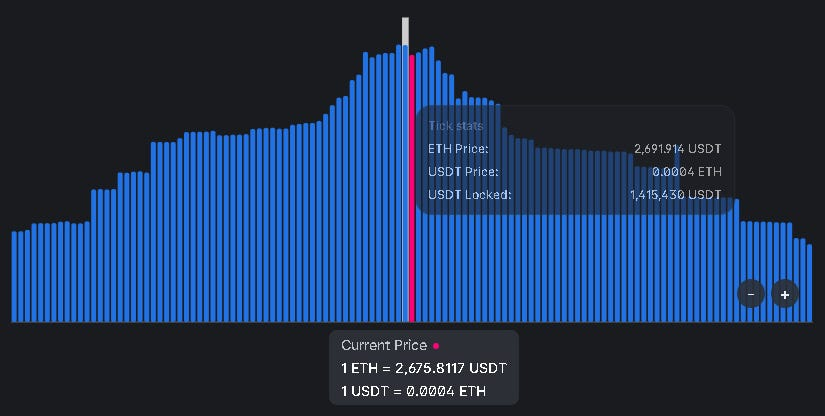Example of liquidity for each price range for the ETH-USDT pool in Uniswap V3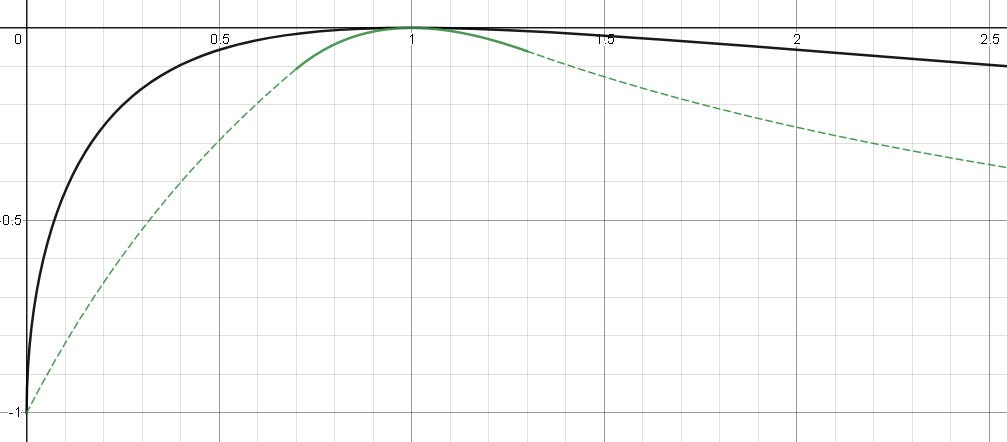Uniswap V3 IL curve in green, V2 IL in black. Dashed line is when the price is out of the range=[0.7, 1.3]

--

--